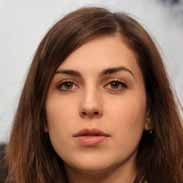# Fall 2013 General Chemistry Final Exam ReviewAlexandra Robertson
question

A chemical can be defined as

any substance that has a definite composition
question

An extensive property of matter is:

volume
question

An intensive property of matter is:

density
question

An intensive property of matter is:

density
question

The two most important properties of all matter are

taking up space and having mass
question

An atom is

the smallest substance that cannot be broken down into simpler, stable substance
question

A compound is

a substance, made of two or more atoms that are chemically bonded, that can be broken down into simpler, stable substance.
question

Physical changes include

grinding, cutting, boiling
question

Chemical changes include

rusting, igniting, burning
question

A chemical change occurs when

a leaf changes color in the fall
question

A physical change occurs when a

glue gun melts a glue stick
question

Noble gases are

unreactive
question

A state a matter with no definite shape and definite volume

liquid
question

A non uniform mixture is

heterogeneous
question

The vertical columns on the periodic table are called

groups
question

The horizontal columns on the periodic table are called

periods
question

Positive charged particle

proton
question

Nuclear particle with same mass as a proton but no charge is a

neutron
question

The nucleus is

positively charged, very dense, and contains nearly all of the mass of the atom.
question

Part of the atom that has a mass 1/2000 of the mass of the hydrogen atom is

the electron
question

Protons are attracted by

the nuclear force
question

An atom is electrically neutral because

the numbers of protons and electrons are equal
question

Most of the volume of the atom is occupied by

electrons
question

The charge due to the electrons of a neutral atom

balances the charge on the nucleus
question

The smallest unit of an element can exist is the

atom
question

Isotopes are atoms of the same element that have different

masses
question

The most common form of hydrogen has

no neutrons
question

The tritium atom consists of

1 proton, 2 neutrons, 1 electron
question

The mass number of deuterium is

2
question

How many isotopes of Hydrogen are there?

3
question

Protons + Neutrons is the

mass number
question

As the mass number of an elements isotopes of an element increases, the number of protons

remains the SAME.
question

All atoms of the same element have the same

Atomic number
question

Elements 90-103 are called the

Actinids
question

Elements 58-71 are called the

Lanthanides
question

Helium, Neon, Argon, Kryton, Xenon, and Radon are

Noble gases
question

What are shared in a covalent bond?

electrons
question

Nuclear forces exist because the particles in the nucleus are

close together
question

All isotopes of hydrogen contain

1 proton
question

The atomic number of Oxygen is 8 because it has

8 protons
question

As the atomic number increases the number of electrons in a NEUTRAL atom